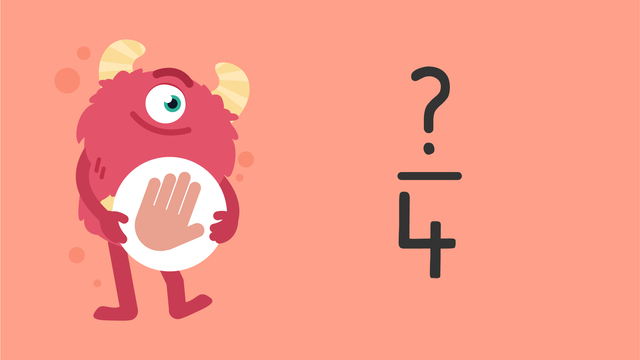# Fraction Word Problems—Let's Practice!Rating

Give us a rating!
The authorsTeam Digital

## Basics on the topicFraction Word Problems—Let's Practice!

Today we are solving fraction word problems with Razzi! This video contains examples to help you further practice and grow confident in this topic.

### TranscriptFraction Word Problems—Let's Practice!

Razzi says get these items ready (...) Because today we're going to practice... Fraction Word Problems. It's time to begin! Four racoons live in a tree. Two are wearing blue bow ties, one is wearing a red scarf, and one is wearing a pink hair bow. What fraction of the racoons are wearing blue bow ties? Pause the video to work on the problem (...) and press play when you are ready to see the solution! Since two out of four racoons are wearing blue bow ties... the answer is two-fourths! Did you also get two-fourths? Let's tackle the next problem! Nine animals live in the savanna. There are three elephants, five flamingos, and one lion. What fraction of the animals are flamingos? Pause the video to work on the problem (...) and press play when you are ready to see the solution! Since five out of nine animals are flamingos... the answer is five-ninths! Did you also get five-ninths? Let's tackle the final problem! Twelve animals live on a farm. There are three horses, two donkeys, three chickens, and four piglets. What fraction of the farm animals are donkeys? Pause the video to work on the problem (...) and press play when you are ready to see the solution! Since two out of twelve animals are donkeys... the answer is two-twelfths! Did you also get two-twelfths? Razzi had so much fun practicing with you today! See you next time!

## Fraction Word Problems—Let's Practice! exercise

Would you like to apply the knowledge you’ve learned? You can review and practice it with the tasks for the video Fraction Word Problems—Let's Practice!.
• ### What fraction of the animals are lions?

Hints

When finding the fraction that are lions, the numerator, or the top number, is how many lions there are.

The denominator, or the bottom number, is how many animals there are in total.

Solution

In total there are 9 animals. 4 of the animals are lions.

The fraction that are lions is $\mathbf{\frac{4}{9}}$.

• ### Fractions of candy.

Hints

When finding the fraction of candies that are red, to find the numerator, count how many red pieces of candy there are.

To find the denominator, look at how many candies there are altogether.

Solution
• There are 7 pieces of candy in total. 4 of them are yellow, 1 is blue and 2 pieces of candy are red.
• There are 7 pieces of candy in total.
• The fraction of candies that are red is $\mathbf{\frac{2}{7}}$.
• ### What fraction are ladybugs?

Hints

The numerator (top part of the fraction) is where we write the number of ladybugs.

The denominator (bottom part of the fraction) is where we write how many insects there are altogether.

Solution

There are 3 ladybugs out of 10 insects in total, so we write this as $\mathbf{\frac{3}{10}}$.

• ### Fractions of flowers.

Hints

When writing the fraction of flowers, the numerator is the number of that individual flower when counted up.

The denominator is the number of flowers there are altogether.

When all of the flowers are counted up, there are 17 in total.

Solution
• When we count all of the flowers, there are 17 in total, so this is always the denominator.
• There are 6 tulips out of 17 flowers: $\frac{6}{17}$.
• There are 5 daisies out of 17 flowers: $\frac{5}{17}$.
• There are 6 daffodils out of 17 flowers: $\frac{6}{17}$.
• ### What fraction are strawberries?

Hints

First, count how many strawberries there are, this is the numerator or the top part of the fraction.

Then, count how many pieces of fruit there are altogether, this is the denominator or the bottom part of the fraction.

Solution

$\mathbf{\frac{5}{7}}$ of the pieces of fruit are strawberries.

This is because there are 7 pieces of fruit altogether and 5 of them are strawberries.

We write 5 out of 7 as $\mathbf{\frac{5}{7}}$.

• ### Solve the big cat word problems.

Hints

Four out of twelve cats are lions. How can this be simplified?

To simplify a fraction, divide both the numerator and denominator by the same factor. What is the largest number that both 6 and 12 can be divided by?

Solution

One third of the cats are lions. We know this because there are twelve big cats in total and four of them are lions.

Cheetahs and leopards make up $\frac{6}{12}$ of all the big cats, in its simplest form we write this as half or $\frac{1}{2}$ .